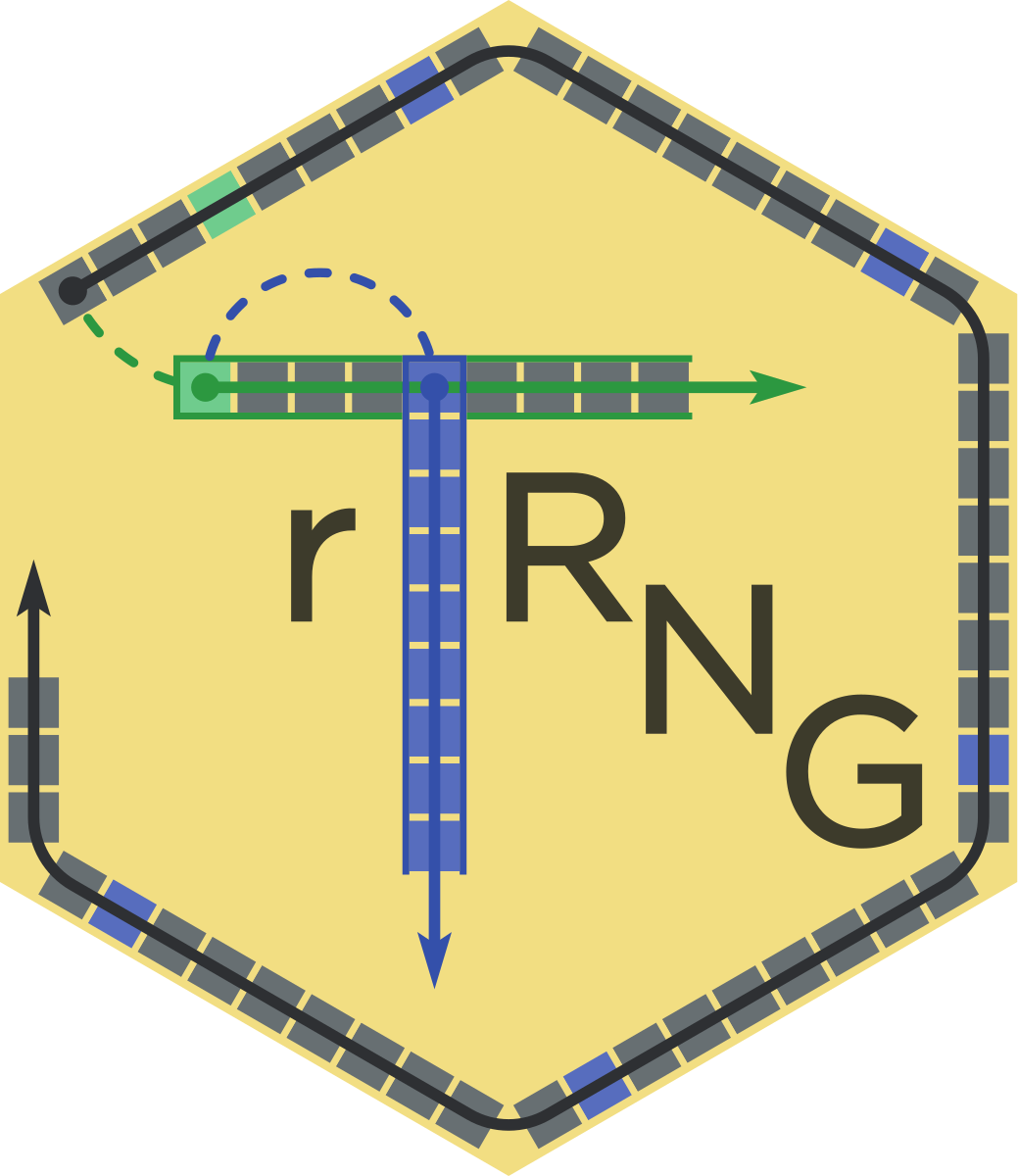# rTRNG: ADVANCED PARALLEL RNG IN R

Following on the recent CRAN release of rTRNG, it’s time to show its features and usage more in detail.rTRNG is a new package for advanced parallel Random Number Generation in R. It relies on TRNG (Tina’s Random Number Generator), a state-of-the-art C++ pseudo-random number generator library for sequential and parallel Monte Carlo simulations. In particular, parallel random number generators provided by TRNG can be manipulated by jump and split operations. These allow to jump ahead by an arbitrary number of steps and to split a sequence into any desired sub-sequence(s), thus enabling techniques such as block-splitting and leapfrogging suitable to parallel algorithms.

#### Background and Motivation

Monte Carlo simulations provide a powerful computational approach to address a wide variety of problems in several domains, such as physical sciences, engineering, computational biology and finance. The independent-samples and large-scale nature of Monte Carlo simulations make the corresponding computation suited for parallel execution, at least in theory. In practice, pseudo-random number generators (RNGs) are intrinsically sequential. This often prevents having a parallel Monte Carlo algorithm that is playing fair, meaning that results are independent of the architecture, parallelization techniques and number of parallel processes.

#### Advanced Parallel RNG in R

rTRNG provides the R user with access to the functionality of the underlying TRNG C++ library. It makes use of Rcpp and RcppParallel to expose the creation, manipulation and use of pseudo-random streams to R. Moreover, the TRNG library and its headers are made available to other projects combining R with C++.

An introduction to rTRNG (pdf) was given at the useR!2017 conference, and is also available as vignette("rTRNG.useR2017", "rTRNG"). A second vignette, vignette("mcMat", "rTRNG"), shows rTRNG in action for the flexible and consistent (parallel) simulation of a matrix of Monte Carlo variates, represented in the picture above.

The concrete application of such techniques to the simulation of credit defaults was presented at the R/Finance 2017 conference, showing how rTRNG can be used for fast sub-portfolio simulation, risk-insight and scenario assessment. The code and data underlying this applied use-case are hosted on GitHub, as is the corresponding R Markdown output.

Below we illustrate, using simple examples, the several ways rTRNG can be used.

##### Base-R-like usage

Similar to base-R (see ?Random), rTRNG allows to select and manipulate a current TRNG generator of a given kind (e.g. yarn2), and to draw from it using any of the provided r<dist>_trng functions:

library(rTRNG)
TRNGkind("yarn2")
TRNGseed(12358)
runif_trng(15)
##   0.580259813 0.339434026 0.221393682 0.369402388 0.542678773
##   0.002851459 0.123996486 0.346813776 0.121799416 0.947124450
##  0.336516569 0.128926181 0.380379891 0.550692382 0.436002654


The special jump and split operations can be applied to the current generator in a similar way:

TRNGseed(12358)
TRNGjump(6) # advance by 6 the internal state
TRNGsplit(5, 3) # generate one element every 5 starting from the 3rd
runif_trng(2)
##  0.1217994 0.5506924
#   => compare to the full sequence above

##### Direct manipulation of generators

Reference objects wrapping the underlying C++ TRNG generators can be created and manipulated in OOP-style, for greater flexibility in using parallel RNGs in R:

rng <- yarn2$new() rng$seed(12358)
rng$jump(6) rng$split(5, 3)
runif_trng(2, engine = rng)
##  0.1217994 0.5506924

##### Parallel generation

Fair-playing, multi-threaded generation of random variates from R can be anabled in r<dist>_trng via argument parallelGrain > 0, where the number of parallel threads is controlled via RcppParallel::setThreadOptions():

TRNGseed(12358)
x_parallel <- runif_trng(1e5, parallelGrain = 100)
TRNGseed(12358)
x_serial <- runif_trng(1e5)
identical(x_serial, x_parallel)
##  TRUE

##### Standalone C++ code

The TRNG C++ library is made available by rTRNG to standalone C++ code compiled with Rcpp::sourceCpp() thanks to the Rcpp::depends attribute:

// [[Rcpp::depends(rTRNG)]]
#include <Rcpp.h>
#include <trng/yarn2.hpp>
#include <trng/uniform_dist.hpp>
// [[Rcpp::export]]
Rcpp::NumericVector exampleCpp() {
trng::yarn2 rng(12358);
rng.jump(6);
rng.split(5, 2); // 0-based index
Rcpp::NumericVector x(2);
trng::uniform_dist<>unif(0, 1);
for (unsigned int i = 0; i < 2; i++) {
x[i] = unif(rng);
}
return x;
}

exampleCpp()
##  0.1217994 0.5506924

##### R packages

Creating an R package with C++ code using the TRNG library via rTRNG is achieved by

• adding Imports: rTRNG and LinkingTo: rTRNG to the DESCRIPTION file

• importing one symbol in the NAMESPACE: importFrom(rTRNG, TRNG.Version)

• setting the relevant linker flags in Makevars[.win] via rTRNG::LdFlags()

• Makevars: PKG_LIBS += $(shell${R_HOME}/bin/Rscript -e "rTRNG::LdFlags()")
• Makevars.win: PKG_LIBS += $(shell "${R_HOME}/bin\${R_ARCH_BIN}/Rscript.exe" -e "rTRNG::LdFlags()")